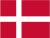### Dish Cloth 56

Cast on 60 stitches and knit 4 rows and then pattern till the cloth has the desired length. Knit 4 rows and bind off.

Pattern:

1.row: 1 p, 1 k, 2 p, 1 k, 2 p, 1 k, 1 p, 1 k
2.row: 1 p, 2 k, 1 p, 1 k, 1 p, 1 k, 1 p, 2 k
3.row: 3 p, 3 k, 3 p, 1 k
4.row: 1 p, 4 k, 1 p, 4 k
5.row: as row 3
6.row: as row 2
7.row: as row 1
8.row: 2 p, 3 k, 1 p, 3 k, 1 p
9.row: 4 p, 1 k, 4 p, 1 k
10.row: as row 8

 X X X X X X 10 X X X X X X X X 9 X X X X X X 8 X X X X X X 7 X X X X X X 6 X X X X X X 5 X X X X X X X X 4 X X X X X X 3 X X X X X X 2 X X X X X X 1

You have to remember that all uneven rows start in the right side of the diagram and X=purl and ▢= knit. All equal rows start in the left side of the diagram and X=knit and ▢=purl.Danish